# Analyze GPS Satellite Visibility

This example shows how to simulate and analyze GPS satellite visibility at specified receiver positions and times using a GPS ephemeris or almanac file. Use the live script controls to set various parameters for the satellite simulation.

### Specify Simulation Parameters

Specify the navigation message file type, start time, duration in hours, and time between samples in seconds of the simulation. Also, specify the position of the receiver in geodetic coordinates and the mask angle, or minimum elevation angle, of the receiver.

```gnssFileType =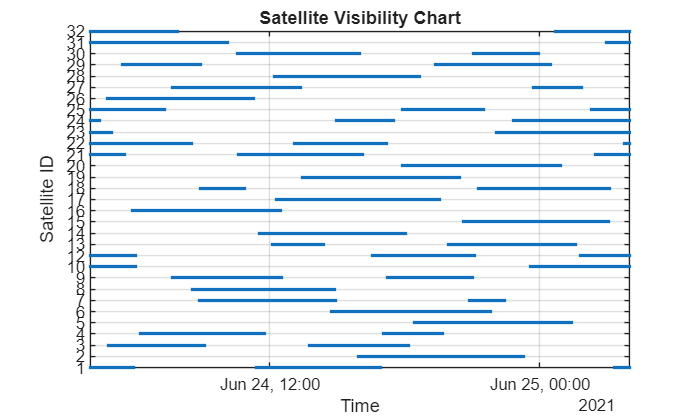"RINEX"; startTime = datetime(2021,06,24,04,00,00); numHours =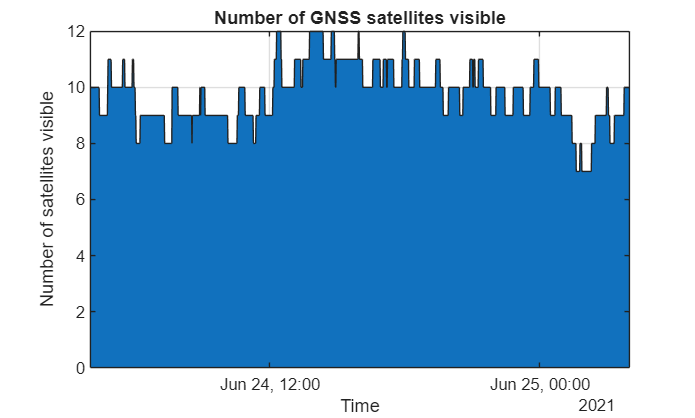24; dt =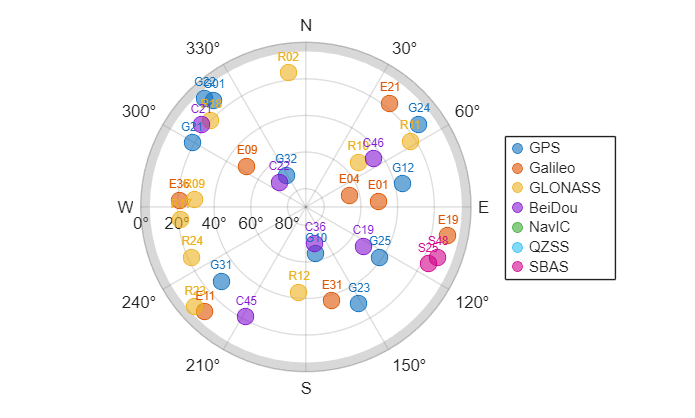60; % s latitude =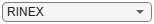42.3013162; % deg longitude =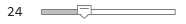-71.3782972; % deg altitude =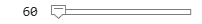50; % m recPos = [latitude longitude altitude]; % [deg deg m] maskAngle =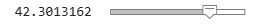5; % deg```

### Get Satellite Orbital Parameters

Use the `exampleHelperParseGNSSFile` helper function to obtain the initial satellite orbital parameters and satellite IDs from a GNSS file. Because ephemeris data is valid for two hours before and after the time of ephemeris, you can use it to determine the exact positions of the satellites in orbit. In contrast, while almanac data, is valid for a period of 90 days, you can only use it for estimating the approximate positions of the satellites.

`[navmsg,satIDs] = exampleHelperParseGNSSFile(gnssFileType);`

The files used in this example are tied to the specified start time `startTime`. To use different RINEX files, use the RINEX files included in the Read Navigation and Observation Data from RINEX File example. For examples of how to download and use different SEM and YUMA almanac files, see the Read GPS Navigation Message Data from SEM Almanac File and Read GPS Navigation Message Data from YUMA Almanac File, respectively.

Once you have new ephemeris data or an almanac file, update the `file` variable of the `exampleHelperParseGNSSFile` helper function for the case that corresponds to your file type.

### Generate Satellite Visibilities

Using your simulation parameters, generate the satellite visibilities as a matrix of logical values. Each row in the matrix corresponds to a time step, and each column corresponds to a satellite. To plot visibilities, iterate through the time vector while calculating the satellite positions and look angles based on the GNSS constellation simulation.

```secondsPerHour = 3600; timeElapsed = 0:dt:(secondsPerHour*numHours); t = startTime+seconds(timeElapsed); numSats = numel(satIDs); numSamples = numel(t); az = zeros(numSamples,numSats); el = zeros(numSamples,numSats); vis = false(numSamples,numSats); sp = skyplot([],[],MaskElevation=maskAngle); for ii = 1:numel(t) satPos = gnssconstellation(t(ii),navmsg,GNSSFileType=gnssFileType); [az(ii,:),el(ii,:),vis(ii,:)] = lookangles(recPos,satPos,maskAngle); set(sp,AzimuthData=az(ii,vis(ii,:)), ... ElevationData=el(ii,vis(ii,:)), ... LabelData=satIDs(vis(ii,:))) drawnow limitrate end```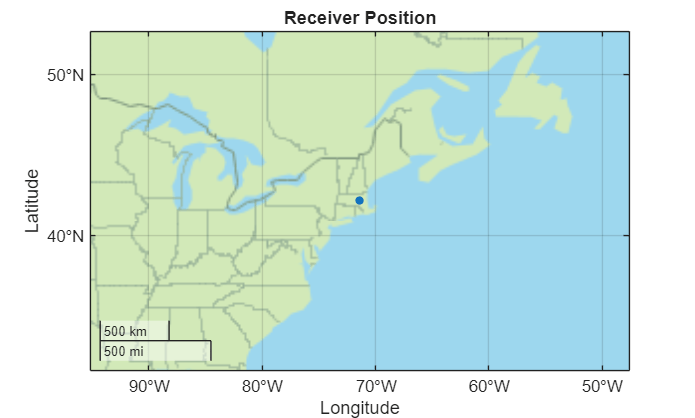### Plot Results

Use the logical matrix to generate a satellite visibility chart, and plot the total number of visible satellites at each time step. In general, at least four satellites must be visible to compute a positioning solution.

```visPlotData = double(vis); visPlotData(visPlotData == false) = NaN; % Hide invisible satellites. visPlotData = visPlotData + (0:numSats-1); % Add space to satellites to be stacked. colors = colororder; figure plot(t,visPlotData,".",Color=colors(1,:)) yticks(1:numSats) yticklabels(string(satIDs)) grid on ylabel("Satellite ID") xlabel("Time") title("Satellite Visibility Chart") axis tight```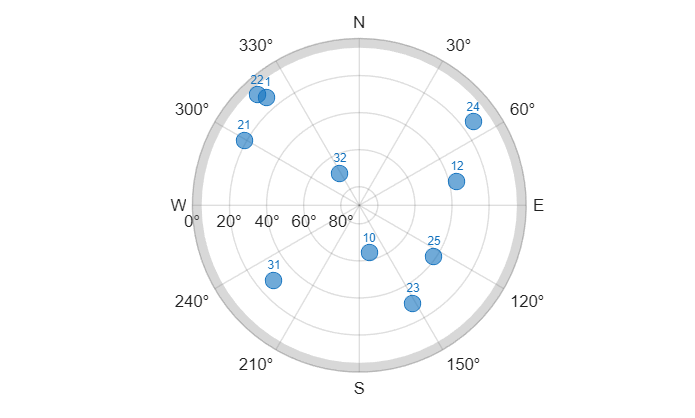```numVis = sum(vis,2); figure area(t,numVis) grid on xlabel("Time") ylabel("Number of satellites visible") title("Number of GPS satellites visible") axis tight```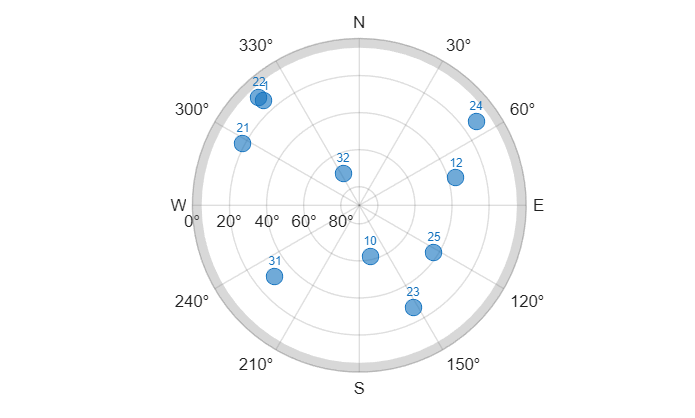### Helper Function

Use the `exampleHelperParseGNSSFile` helper function to obtain the initial satellite orbital parameters and satellite IDs from the GNSS file, based on the file type.

```function [navmsg,satIDs] = exampleHelperParseGNSSFile(gnssFileType) switch gnssFileType case "RINEX" file = "GODS00USA_R_20211750000_01D_GN.rnx"; navmsg = rinexread(file); % For RINEX files, extract gps data and use only the first entry for each satellite. gpsData = navmsg.GPS; [~,idx] = unique(gpsData.SatelliteID); navmsg = gpsData(idx,:); satIDs = navmsg.SatelliteID; case "SEM" file = "semalmanac_2021-6-22.al3"; navmsg = semread(file); satIDs = navmsg.PRNNumber; case "YUMA" file = "yumaalmanac_2021-6-22.alm"; navmsg = yumaread(file); satIDs = navmsg.PRN; end end```